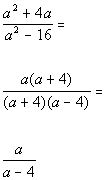TitleCollege Algebra
Answer/Discussion to Practice Problems:
Tutorial 8: Simplifying Rational ExpressionsAnswer/Discussion to 1aOur restriction is that the denominator of a fraction can never be equal to 0.  So to find what values we need to exclude, think of what value(s) of x, if any, would cause the denominator to be 0.*Factor the den.

 This give us a better look at it.  Since 0 would make the first factor in the denominator 0, then 0 would have to be excluded. Since 4 would make the second factor in the denominator 0, then 4 would also have to be excluded.Answer/Discussion to 2aStep 1: Factor the numerator and the denominator AND

 Step 2: Divide out all common factors that the numerator and the denominator have.*Factor the GCF in the num. and  *Factor the diff. of squares in the den.  *Divide out the common factor of (a + 4) *Rational expression simplified

 To find the value(s) needed to be excluded from the domain, we need to ask ourselves, what value(s) of a would cause our denominator to be 0? Looking at the denominator a - 4, I would say it would have to be a = 4.  Don't you agree? 4 would be our excluded value.Answer/Discussion to 2bStep 1: Factor the numerator and the denominator AND

 Step 2: Divide out all common factors that the numerator and the denominator have.*Factor the trinomial in the den.   *Factor out a -1 from (8 - x)   *Divide out the common factor of (x - 8) *Rational expression simplified

 Note that 8 - x and x - 8 only differ by signs, in other words they are opposites of each other.  In that case, you can factor a -1 out of one of those factors and rewrite it with opposite signs, as shown in line 3 above.   To find the value(s) needed to be excluded from the domain, we need to ask ourselves, what value(s) of x would cause our denominator to be 0? Looking at the denominator x + 1, I would say it would have to be x = -1.  Don't you agree? -1 would be our excluded value.

Last revised on Dec. 14, 2009 by Kim Seward.
All contents copyright (C) 2002 - 2010, WTAMU and Kim Seward. All rights reserved.